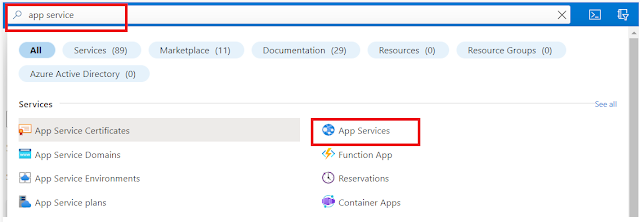### HackerRank Python Solution - Sets - .union() Operation

.union():
• The .union() operator returns the union of a set and the set of elements in an iterable. Sometimes, the | operator is used in place of .union() operator, but it operates only on the set of elements in set.
• Set is immutable to the .union() operation (or | operation).

Example:

``````>>> s = set("Hacker")
>>> print s.union("Rank")
set(['a', 'R', 'c', 'r', 'e', 'H', 'k', 'n'])

>>> print s.union(set(['R', 'a', 'n', 'k']))
set(['a', 'R', 'c', 'r', 'e', 'H', 'k', 'n'])

>>> print s.union(['R', 'a', 'n', 'k'])
set(['a', 'R', 'c', 'r', 'e', 'H', 'k', 'n'])

>>> print s.union(enumerate(['R', 'a', 'n', 'k']))
set(['a', 'c', 'r', 'e', (1, 'a'), (2, 'n'), 'H', 'k', (3, 'k'), (0, 'R')])

>>> print s.union({"Rank":1})
set(['a', 'c', 'r', 'e', 'H', 'k', 'Rank'])

>>> s | set("Rank")
set(['a', 'R', 'c', 'r', 'e', 'H', 'k', 'n'])
``````
• The students of District College have subscriptions to English and French newspapers. Some students have subscribed only to English, some have subscribed to only French and some have subscribed to both newspapers.
• You are given two sets of student roll numbers. One set has subscribed to the English newspaper, and the other set is subscribed to the French newspaper. The same student could be in both sets. Your task is to find the total number of students who have subscribed to at least one newspaper.
Input Format:
• The first line contains an integer, n, the number of students who have subscribed to the English newspaper.
• The second line contains n space separated roll numbers of those students.
• The third line contains b, the number of students who have subscribed to the French newspaper.
• The fourth line contains b space separated roll numbers of those students.
Constraints:
• 0 < Total number of students in college < 1000
Output Format:
• Output the total number of students who have at least one subscription.
Sample Input:

``````9
1 2 3 4 5 6 7 8 9
9
10 1 2 3 11 21 55 6 8
``````
Sample Output:

``````13
``````
Explanation:

Roll numbers of students who have at least one subscription:
• 1, 2, 3, 4, 5, 6, 7, 8, 9, 10, 11, 21 and 55. Roll numbers: 1, 2, 3, 6 and 8 are in both sets so they are only counted once.
• Hence, the total is 13 students.
Solution:

``````m = int(input())

eng_roll = set(input().split())

n = int(input())

french_roll = set(input().split())

print(len(eng_roll.union(french_roll)))
``````
Disclaimer: The problem statement is given by hackerrank.com but the solution is generated by the Geek4Tutorial admin. If there is any concern regarding this post or website, please contact us using the contact form. Thank you!